## A parallel plate capacitor with plate area 4.0 cm2 and air gap separation 0.50 mm is connected to a 12-V battery, and fully charged. The bat

Question

A parallel plate capacitor with plate area 4.0 cm2 and air gap separation 0.50 mm is connected to a 12-V battery, and fully charged. The battery is then disconnected.
(a) What is the charge on the capacitor?
(b) The plates are now pulled to a separation of 1.00 mm. What is the charge on the capacitor now?

(c) What is the potential difference across the plates now?

(d) How much work was required to pull the plates to their new separation?

in progress 0
2 months 2021-07-29T16:07:19+00:00 1 Answers 6 views 0

(a) The charge on capacitor in first case is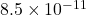C

(b) In second case is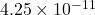C

(c) Potential across plate is 24 V

(d) Work required to pull the plates is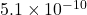J

Explanation:

Given:

Area of plate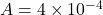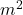Voltage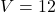V

Separation between two plate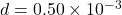m

(a)

The charge on capacitor is given by,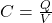But capacitance of parallel plate capacitor is given by,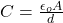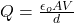Where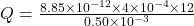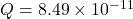C

(b)

The charge on the capacitor when plate separation is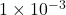m is,

Here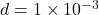m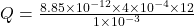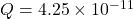C

(c)

The potential difference across plate is,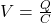But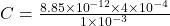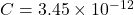F

Put the value of capacitance and find potential difference,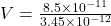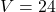V

(d)

Work required is given by,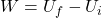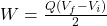Where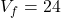V and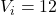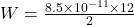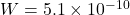J

Therefore, the charge on capacitor in first case isC and in second case isC and potential across plate is 24 V and work required to pull the plates isJ